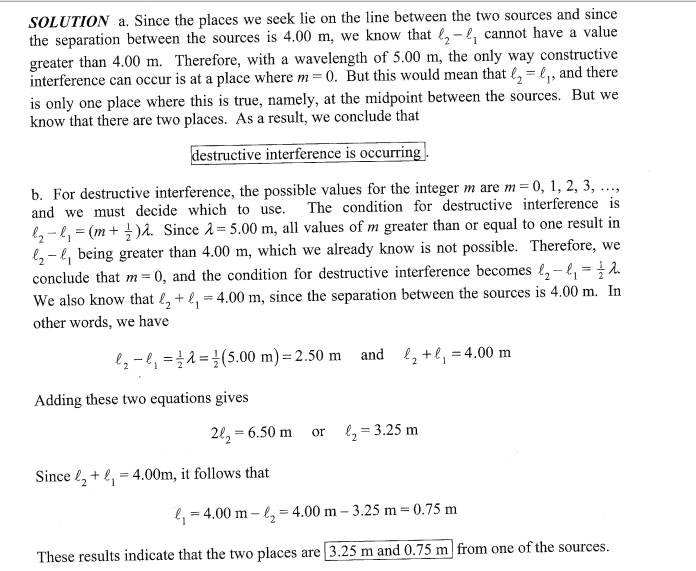# Interference and wave nature of light

Two in-phase sources of waves are separated by a distance of 4.00 m. These sources produce identical waves that have a wavelength of 5.00 m. On the line between them, there are two places at which the same type of interference occurs. Where are the places located?

I already know the answers from this website http://lev.ccny.cuny.edu/~hmakse/TEACHING/204soln27.pdf [Broken] problem 3. However I cannot for the life of me understand the solution. I especially don't understand why d1 + d2 has to be less than 4. I have spent an hour on this problem and re-reading the explanation and my textbook. But it just doesn't click.

Last edited by a moderator:

haruspex
Homework Helper
Gold Member
2020 Award
I followed that link, but unfortunately some of the pages appear blank for me, including the one with the relevant solution. Are d1 and d2 the distances from a particular source? If so, let F1, f2 be the distances from the other source. You know d1 + d2 + f1 +f2 = 8m, so either d1 + d2 <= 4m or f1+f2 <= 4m. So choose as reference the source which is nearer on average.Here is the solution. I think I am having trouble envisioning the scenario :/

haruspex